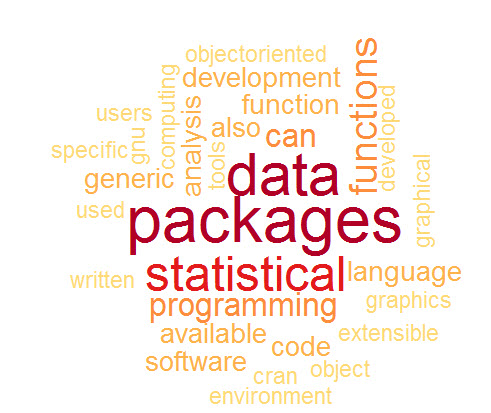# R: Text Mining (Pre-processing)

This is part 2 of my Text Mining Lesson series. If you haven’t already, please check out part 1 that covers Term Document Matrix: R: Text Mining (Term Document Matrix)

Okay, now I promise to get to the fun stuff soon enough here, but I feel that in most tutorials I have seen online, the pre-processing of text is often glanced over. It was always (and often still is) a real sore spot for me when assumptions are made as to my knowledge level. If you are going to throw up a block of code, at least give a line or two explanation as to what the code is there for, don’t just assume I know.

I remember working my way through many tutorials where I was able to complete the task by simply copying the code, but I didn’t have a full grasp of what was happening in the middle. I this lesson, I am going to cover some of the more common text pre-processing steps used in the TM library. I am going to go into some level of detail and make some purposeful mistakes so hopefully when you are done here you will have a firm grasp on this very important step in the text mining process.

Let’s start by getting our libraries

```install.packages("tm") # if not already installed
install.packages("SnowballC")

library(tm)
library(SnowballC)```

Now, let’s load our data. For this lesson we are going to use a simple vector.

```wordVC <- c("I like dogs!", "A cat chased the dog.", "The dog ate a bone.",
"Cats make fun pets.", "The cat did what? Don't tell the dog.",
"A cat runs when it has too, but most dogs love running!")```

Now let’s put this data into a corpus for text processing.

```corpus <- (VectorSource(wordVC))
corpus <- Corpus(corpus)
summary(corpus)```

Here is the outputNow for a little frustration. Let’s say you want to see what is in text document 4. You could try

`inspect(corpus)`

But this will be your output, not really what you are looking for.If you want to see the actual text- try this instead

`corpus[]\$content`

Now you can see the textAs we go through the text pre-processing, we are going to use the following For Loop to examine our corpus

`for (i in 1:6) print (corpus[[i]]\$content)`

Output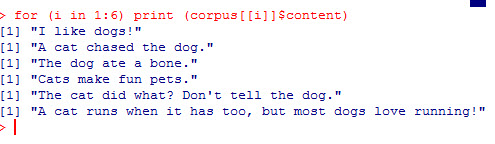### Punctuation

Punctuation generally adds no value to text mining when utilizing standard numeric based data mining algorithms like clustering or classification. So it behooves us to just remove it.

To do so, the tm package has a cool function called tm_map() that we can pass arguments to, such as removePunctuation

``````corpus <- tm_map(corpus, content_transformer(removePunctuation))

```for (i in 1:6) print (corpus[[i]]\$content)```

Note, you do not need the for loop, I am simply running it each time to show you the progress.

Notice all the punctuation is gone now.### Stopwords

Next we are going to get rid of what are known as stopwords. Stopwords are common words such as (the, an, and, him, her). These words are so commonly used that they provide little insight as to the actual meaning of the given text.  To get rid of them, we use the following code.

```corpus <- tm_map(corpus, content_transformer(removeWords),
stopwords("english"))
for (i in 1:6) print (corpus[[i]]\$content)```

If you look at line 2, “A cat chase  dog”, you will the word “the” has been removed. However, if you look at the next line down, you will notice “The” is still there.WHY?

Well it comes down to the fact that computers do not treat T and t as the same letter, even though they are. Capitalized letters are viewed by computers as separate entities. So “The” doesn’t match “the” found in the list of stopwords to remove.

For a full list of R stopwords, go to: https://github.com/arc12/Text-Mining-Weak-Signals/wiki/Standard-set-of-english-stopwords

So how do we fix this?

### tolower

Using tm_map with the “tolower” argument will make all the letters lowercase. If we then re-run our stopwords command, you will see all the “the” are gone

```corpus <- tm_map(corpus, content_transformer(tolower))
corpus <- tm_map(corpus, content_transformer(removeWords),
stopwords("english"))
for (i in 1:6) print (corpus[[i]]\$content)```

Output### stemming

Next we will stem our words. I covered this in the last lesson, but it bears repeating. What stemming does is attempt to remove variants of words. In our example, pay attention to the following words (dog, dogs, cat, cats, runs, running)

```corpus <- tm_map(corpus, stemDocument)
for (i in 1:6) print (corpus[[i]]\$content)```

Notice the words are now (dog, cat, run)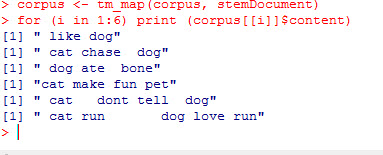### Whitespace

Finally, let’s get rid of all this extra white space we have now.

``````corpus <- tm_map(corpus, stripWhitespace)
for (i in 1:6) print (corpus[[i]]\$content)``````

Output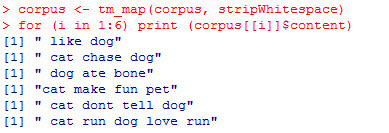### removeNumbers

I didn’t use this argument with my tm_map() function today because I did not have any numbers in my text. But if I, the command would be as follows

`corpus <- tm_map(corpus, content_transformer(removeNumbers))`

# R: Text Mining (Term Document Matrix)

There are a bounty of well known machine learning algorithms, both supervised (Decision Tree, K Nearest Neighbor, Logistical Regression) and unsupervised (clustering, anomaly detection). The only catch is that these algorithms are designed to work with numbers, not text. The act of using numeric based data mining methods on text is known as duo-mining.

So before you can utilize these algorithms, you first have to  transform text into a format suitable for use in these number based algorithms. One of the most popular methods people first learn is how to create a Term Document Matrix (TDM). The easiest way to understand this concept is, of course, to do an example.

```install.packages("tm") # if not already installed
library(tm)```

Now let’s create a simple vector of strings.

```wordVC <- c("I like dogs", "A cat chased the dog", "The dog ate a bone",
"Cats make fun pets")```

Now we are going to place the strings into a data type designed for text mining (from the tm package) called corpus. A corpus simply means the full collection of text you want to work with.

```corpus <- (VectorSource(wordVC))
corpus <- Corpus(corpus)
summary(corpus)```

Output:

``` Length Class Mode
1 2 PlainTextDocument list
2 2 PlainTextDocument list
3 2 PlainTextDocument list
4 2 PlainTextDocument list```

As you can see from the summary, the corpus classified each string in the vector as a PlaintextDocument.

Now let’s create our first Term Document Matrix

```TDM <- TermDocumentMatrix(corpus)
inspect(TDM)```

Output: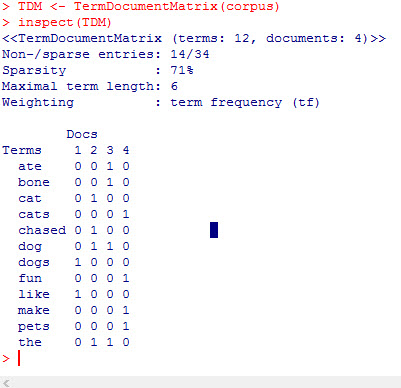As you can see, we now have a numeric representation of our text. Each row represents a word in our text and each column represents an individual sentence. So if you look at the word dog, you can see it appears once in sentence 2 and 3, (0110). While bone appears once in sentence 3 only (0010).

## Stemming

One immediate issue that jumps out at me is that R now sees the words cat & cats and dog & dogs as different words, when really cats and dogs are just the plural versions of cat and dog. Now there may be some more advanced applications of text mining that you would want to keep the two words separate, but in most basic text mining applications, you would want to only keep one version of the word.

Luckily for us, R makes that simple. Use the function tm_map with the argument stemDocument

`corpus2 <- tm_map(corpus, stemDocument)`

make a new TDM

```TDM2 <- TermDocumentMatrix(corpus2)
inspect(TDM2)```

Now you see only the singular of cat and dog exist in our list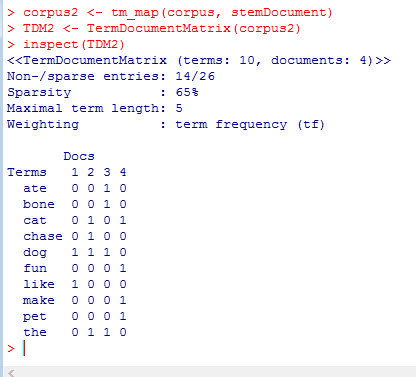If you would like, you can also work with the transpose of the TDM called the Document Term Matrix.

```dtm = t(TDM2)
inspect(dtm)```

dfsaI’ll get deeper into more pre-processing tasks, as well as ways to work with your TDM in future lessons. But for now, practice making TDMs see if you can think of ways that you can use TDMs and DTMs with some machine learning algorithms you might already know (decision trees, logistic regression).

# R: Creating a Word Cloud

Word Clouds are great visualization techniques for dealing with text analytics. The idea behind them is they display the most common words in a corpus of text. The more often a word is used, the larger and darker it is.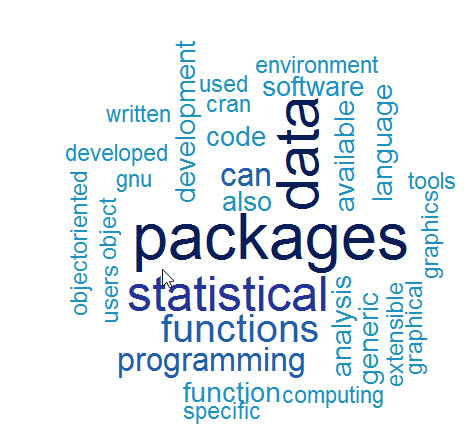Making a word cloud in R is relatively easy. The tm and wordcloud libraries from R’s CRAN repository is used to create one.

```library(tm)
library(wordcloud)

```

If you do not have either of these loaded on your machine, you will have to use the following commands

```install.packages("tm")
install.packages("wordcloud")```

Now in order to make a word cloud, you first need a collection of words. In our example I am going to use a text file I created from the Wikipedia page on R.

Now let’s load the data file.

```text <- readLines("rWiki.txt")
 "R is a programming language and software environment
 "The R language is widely used among statisticians and
 "Polls, surveys of data miners, and studies of scholarly
 "R is a GNU package. The source code for the R
 "General Public License, and pre-compiled binary versions
 "R is an implementation of the S programming language "
>```

Notice each line in the text file is an individual element in the vector –  text

Now we need to move the text into a tm element called a Corpus. First we need to convert the vector text into a VectorSource.

```wc <- VectorSource(text)
wc <- Corpus(wc)```

Now we need to pre-process the data. Let’s start by removing punctuation from the corpus.

`wc <- tm_map(wc, removePunctuation)`

Next we need to set all the letters to lower case. This is because R differentiates upper and lower case letters. So “Program” and “program” would treated as 2 different words. To change that, we set everything to lowercase.

`wc <- tm_map(wc, content_transformer(tolower))`

Next we will remove stopwords. Stopwords are commonly used words that provide no value to the evaluation of the text. Examples of stopwords are: the, a, an, and, if, or, not, with ….

`wc <- tm_map(wc, removeWords, stopwords("english"))`

Finally, let’s strip away the whitespace

`wc <- tm_map(wc, stripWhitespace)`

Now let us make our first word cloud

The syntax is as follows – wordcloud( words = corpus, scale = physical size, max.word = number of words in cloud)

`wordcloud(words = wc, scale=c(4,0.5), max.words=50)`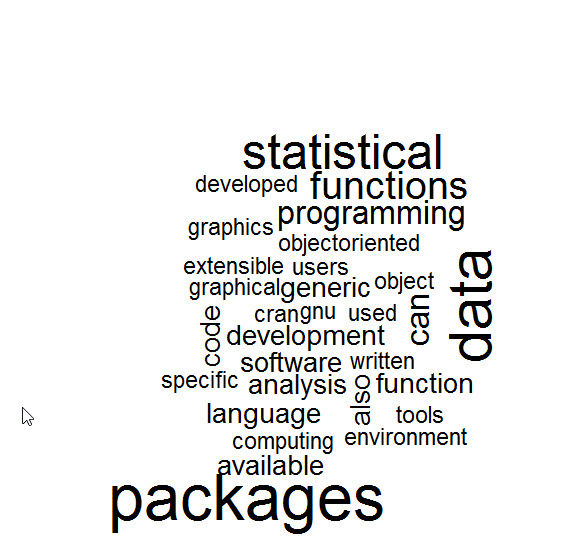Now we have a word cloud, let’s add some more elements to it.

random.order = False brings the most popular words to the center

`wordcloud(words = wc, scale=c(4,0.5), max.words=50,random.order=FALSE)`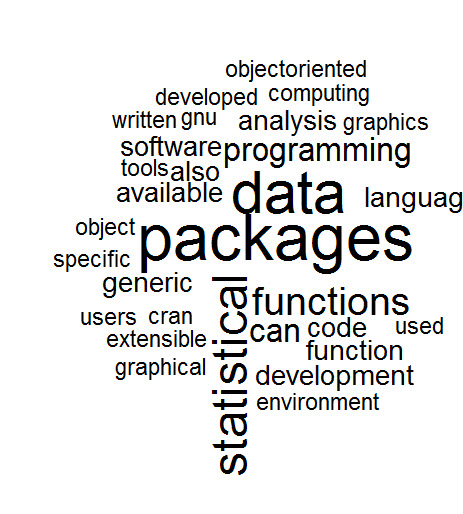To add a little more rotation to your word cloud use rot.per

```wordcloud(words = wc, scale=c(4,0.5), max.words=50,random.order=FALSE,
rot.per=0.25)```

Finally, lets add some color. We are going to use brewer.pal.  The syntax is brewer.pal(number of colors, color mix)

```cp <- brewer.pal(7,"YlOrRd")
wordcloud(words = wc, scale=c(4,0.5), max.words=50,random.order=FALSE,
rot.per=0.25, colors=cp)```